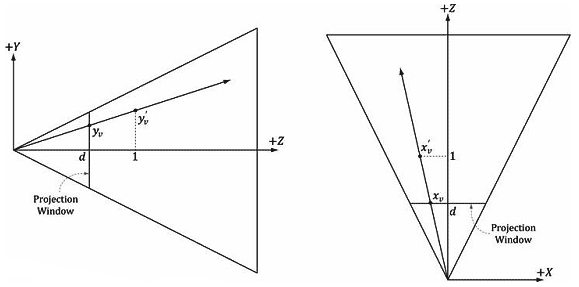# 16.1 屏幕到投影窗口的变换

1．给定屏幕坐标s，求出它在投影窗口上的对应点p

2．在观察空间中计算拾取射线。该射线从观察空间的原点射出，并穿过点p

3．把拾取射线和模型变换到同一个空间，测试模型是否与拾取射线相交。

4．确定与射线相交的物体。（与摄像机距离）最近的物体就是用户拾取的屏幕物体。

${\bf{M}} = \left[ {\begin{array}{*{20}{c}}{\frac{{Width}}{2}}&0&0&0\\0&{ - \frac{{Height}}{2}}&0&0\\0&0&{MaxDepth - MinDepth}&0\\{TopLeftX + \frac{{Width}}{2}}&{TopLeftY + \frac{{Height}}{2}}&{MinDepth}&1\end{array}} \right]$

typedef struct D3D11_VIEWPORT {
FLOAT TopLeftX;
FLOAT TopLeftY;
FLOA Width;
FLOA Height;
FLOAT MinDepth;
FLOAT MaxDepth;
} D3D11_VIEWPORT;

${\bf{M}} = \left[ {\begin{array}{*{20}{c}}{\frac{w}{2}}&0&0&0\\0&{ - \frac{h}{2}}&0&0\\0&0&1&0\\{\frac{w}{2}}&{\frac{h}{2}}&0&1\end{array}} \right]$

$\left[ {{x_{ndc}},{y_{ndc}},{z_{ndc}},1} \right]\left[ {\begin{array}{*{20}{c}}{\frac{w}{2}}&0&0&0\\0&{ - \frac{h}{2}}&0&0\\0&0&1&0\\{\frac{w}{2}}&{\frac{h}{2}}&0&1\end{array}} \right] = \left[ {\frac{{{x_{ndc}}w + w}}{2},\frac{{ - {y_{ndc}}h + h}}{2},{z_{ndc}},1} \right]$

$\begin{array}{l}{x_s} = \frac{{{x_{ndc}}w + w}}{2}\\{y_s} = \frac{{ - {y_{ndc}}h + h}}{2}\end{array}$

$\begin{array}{l}{x_{ndc}} = \frac{{2{x_s}}}{w} - 1\\{y_{ndc}} = - \frac{{2{y_s}}}{h} + 1\end{array}$

$\begin{array}{l} - r \le x' \le r\\ - 1 \le \frac{{x'}}{r} \ge 1\end{array}$

$\begin{array}{l}{x_v} = r\left( {\frac{{2{x_s}}}{w} - 1} \right)\\{y_v} = - \frac{{2{y_s}}}{h} + 1\end{array}$

$\begin{array}{l}{x_v}^\prime = \frac{{{x_v}}}{d} = \frac{{{x_v}}}{{\cos \frac{\alpha }{2}}} = {x_v}\tan \frac{\alpha }{2} = \left( {\frac{{2{x_s}}}{w} - 1} \right)r\tan \frac{\alpha }{2}\\{y_v}^\prime = \frac{{{y_v}}}{d} = \frac{{{y_v}}}{{\cos \frac{\alpha }{2}}} = {y_v}\tan \frac{\alpha }{2} = \left( { - \frac{{2{y_s}}}{h} + 1} \right)\tan \frac{\alpha }{2}\end{array}$图16.4 由相似三角形可知$$\frac{{{y_v}}}{d} = \frac{{{y_v}^\prime }}{1}$$和$$\frac{{{x_v}}}{d} = \frac{{{x_v}^\prime }}{1}$$。

$\begin{array}{l}{x_v}^\prime = \left( {\frac{{2{x_s}}}{w} - 1} \right)/{\bf{P}}{}_{00}\\{y_v}^\prime = \left( { - \frac{{2{y_s}}}{h} + 1} \right)/{{\bf{P}}_{11}}\end{array}$

void PickingApp::pick(int sx, int sy)
{
XMMATRIX P = mCam.Proj();

// 在视空间中计算拾取射线
float vx = (+2.0f*sx/mClientWidth - 1.0f)/P(0,0);
float vy = (-2.0f*sy/mClientHeight + 1.0f)/P(1,1);
// 视空间中的射线定义
XMVECTOR rayOrigin = XMVectorSet(0.0f, 0.0f, 0.0f，1.0f);
XMVECTOR rayDir = XMVectorSet (vx, vy, 1.0f，0.0f);

2006 - 2023，推荐分辨率1024*768以上，推荐浏览器Chrome、Edge等现代浏览器，截止2021年12月5日的访问次数：1872万9823 站长邮箱